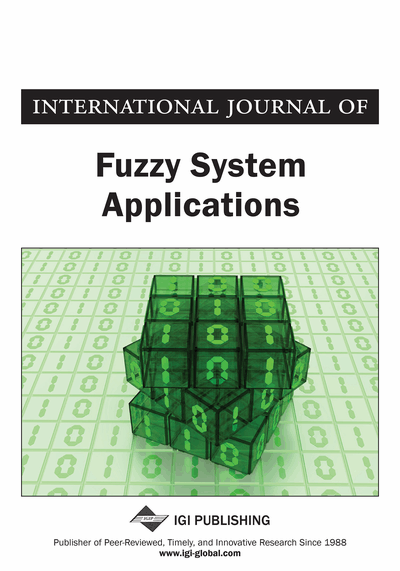# Multi-Fuzzy Complex Nilpotent Matrices

Asit Dey (Department of Applied Mathematics with Oceanology and Computer Programming, Vidyasagar University, Midnapore, India) and Madhumangal Pal (Department of Applied Mathematics with Oceanology and Computer Programming, Vidyasagar University, Midnapore, India)
DOI: 10.4018/IJFSA.2016100103

## Abstract

In this paper, the concept of multi-fuzzy matrix (MFM), multi-fuzzy complex matrix (MFCM), generalized multi-fuzzy complex matrix (GMFCM), generalized multi-fuzzy complex nilpotent matrix (GMFCNM) are introduced and have shown that the set of GMFCMs form a distributive lattice. Some properties and characterizations for GMFCNMs are established and in particular, a necessary and sufficient condition for an n × n GMFCNM to have the nilpotent index n is given. Finally, the reduction of GMFCNMs over a distributive lattice are given with some properties.
Article Preview
Top

## 1. Introduction

The theory of fuzzy sets which was introduced by Zadeh (Zadeh, 1965) is applied to many mathematical branches. Goguen generalized them to the notion of L-fuzzy set (Goguen, 1967). Since then several special L-fuzzy set such as the interval-valued fuzzy set, the intuitionistic fuzzy set, the three-dimensional fuzzy set, the interval-valued intuitionistic fuzzy set and the type-2 fuzzy set have been proposed. Great progress, both in theory and in application, has been made based on these L-fuzzy sets. On the other hand, few years after the inception of the notion of fuzzy set, Rosenfeld (Rosenfeld, 1971) started the pioneer work in the domain of fuzzification of the algebraic objects, with his work on fuzzy group.

With max-min operations the fuzzy algebraand its matrix theory are considered by many authors, see e.g. (Tan, 2001;Tan, 2005;Zhang, 2001) The determinant theory, powers and nilpotent conditions of matrices over a distributive lattice are considered by Zhang (Zhang, 1994, 2001) Tan (Tan, 2001Tan, 2005) and Pal (Pal, 2001).

Algebraic structures play a prominent role in the mathematics with wide range of applications in many disciplines such as theoretical physics, computer science, coding theory, topological spaces, etc. This provides sufficient motivation to the researchers to review various concepts and results from the area of abstract algebra in the broader framework of fuzzy setting. One of the structures which is most extensively discussed in the mathematics and its applications is lattice theory.

A lattice matrix is a matrix whose all entries belong to a distributive lattice. A square lattice matrix A is called nilpotent if Am = 0 for some positive integer m, where 0 is the zero matrix. It is clear that any Boolean matrix and fuzzy matrix are lattice matrices.

Lattice matrices are useful tools in various domains like the theory of switching nets, automata theory, etc. The concept of lattice matrices was introduced by Give'on (Give'on, 1964). The notion of nilpotent lattice matrices have appeared first in the work of Give'on (Give'on, 1964) and Give'on proved that an n × n lattice matrix A is nilpotent if and only if An = 0. After that many researchers have studied the topics of nilpotent lattice matrices. In (Hashimoto, 1982, 1985) Hashimoto considered the reduction of nilpotent fuzzy matrices and obtained some properties of reduction.

Sebastian and Ramakrishnan (Sebastian and Ramakrishnan, 2011) introduced the concepts of multi-fuzzy sets in terms of ordered sequences of membership functions. Buckley (Buckley, 1989)., Rejun, et al. (Rejun, et al., 1985) and others have done some works on fuzzy complex numbers and fuzzy complex sets. The notion of multi-fuzzy complex numbers and multi-fuzzy complex sets is introduced first times by Dey and Pal (Dey and Pal, 2015). Adak et al. (Adak et al., 2012) have done some works on generalized intuitionistic fuzzy nilpotent matrices over distributive lattice.

In recent past, lot of works have done in the topics of fuzzy matrix, intuitionistic fuzzy matrix, interval-valued fuzzy matrix, generalized fuzzy matrix. Motivated from these works we have generalized the previous results on nilpotent fuzzy matrices and reduction of nilpotent matrices proposed by Hashimto (Hashimto, 1985).

## Complete Article List

Search this Journal:
Reset
Open Access Articles: Forthcoming
Volume 9: 4 Issues (2020): 1 Released, 3 Forthcoming
Volume 8: 4 Issues (2019)
Volume 7: 4 Issues (2018)
Volume 6: 4 Issues (2017)
Volume 5: 4 Issues (2016)
Volume 4: 4 Issues (2015)
Volume 3: 4 Issues (2013)
Volume 2: 4 Issues (2012)
Volume 1: 4 Issues (2011)
View Complete Journal Contents Listing